• ## PDE——delta函数

千次阅读 2019-04-08 19:50:35
文章目录$\delta$函数 δ\deltaδ函数 ...它所给出的“函数值”只是在积分运算中才有意义∫−∞∞f(x)δ(x)dx=f(0)\int_{-\infty}^{\infty} f(x) \delta(x) \mathrm{d} x=f(0)∫−∞∞​f(x)δ(x...
文章目录$\delta$函数常微分方程的Green函数常微分方程的初值问题常微分方程的边值问题
$\delta$函数
$\delta$函数，并不是通常意义下的函数：它并没有给出函数与自变量之间的对应关系。
它给出的对应关系在通常意义下是没有意义的
它所给出的“函数值”只是在积分运算中才有意义$\int_{-\infty}^{\infty} f(x) \delta(x) \mathrm{d} x=f(0)$

$\delta$函数也可以理解为（任意阶可微）函数序列的极限。
凡是具有$\lim _{l \rightarrow 0} \int_{-\infty}^{\infty} f(x) \delta_{l}(x) \mathrm{d} x=f(0)$性质的函数序列$\delta_l(x)$，或是具有$\lim _{n \rightarrow \infty} \int_{-\infty}^{\infty} f(x) \delta_{n}(x) \mathrm{d} x=f(0)$性质的函数序列$\delta_n(x)$，他们的极限都是$\delta$函数。
比如：$\delta(x)=\lim _{n \rightarrow \infty} \frac{n}{\sqrt{\pi}} \mathrm{e}^{-n^{2} x^{2}}$
$\delta(x)=\lim _{n \rightarrow \infty} \frac{n}{\pi} \frac{1}{1+n^{2} x^{2}}$
$\delta(x)=\frac{\sin n x}{\pi x}$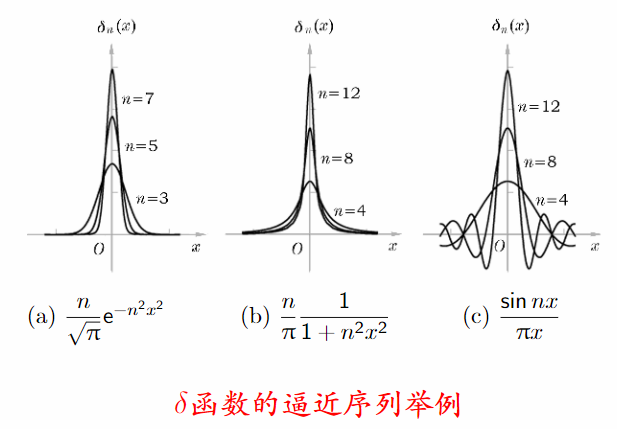------
$\delta$函数的基本运算规则

$\delta$函数和常数c的乘积
\begin{aligned} \int_{-\infty}^{\infty} f(x) c \delta(x) \mathrm{d} x &=\int_{-\infty}^{\infty} c f(x) \delta(x) \mathrm{d} x \\ &=c f(0) \end{aligned}
平移变换，$x \rightarrow x-a$：
$\int_{-\infty}^{\infty} f(x) \delta(x-a) \mathrm{d} x=\int_{-\infty}^{\infty} f(t+a) \delta(t) \mathrm{d} t=f(a)$
放大或缩小，$x \rightarrow \alpha x$：
$\int_{-\infty}^{\infty} f(x) \delta(\alpha x) \mathrm{d} x=\int_{-\infty}^{\infty} f(t / \alpha) \delta(t) \frac{\mathrm{d} t}{|\alpha|}=\frac{1}{|\alpha|} f(0)$
这意味着$\delta(\alpha x)=\frac{1}{|\alpha|} \delta(x)$
特别是$\alpha=-1$时，$\delta(-x)=\delta(x)$
delta函数的导数：对于在x=0点连续并有连续导数的任意函数f(x),有
\begin{aligned} \int_{-\infty}^{\infty} f(x) \delta^{\prime}(x) \mathrm{d} x &=f(x) \delta\left.(x)\right|_{-\infty} ^{\infty}-\int_{-\infty}^{\infty} f^{\prime}(x) \delta(x) \mathrm{d} x \\ &=-f^{\prime}(0) \end{aligned}
这里就把delta函数当作普通的连续函数一样进行分部积分。
delta函数的高阶导数：对于在x=0点连续并有n阶连续导数的任意函数f(x),有
$\int_{-\infty}^{\infty} f(x) \delta^{(n)}(x) \mathrm{d} x=(-)^{n} f^{(n)}(0)$
delta函数与普通函数的乘积，$g(x) \delta(x)$：
\begin{aligned} \int_{-\infty}^{\infty} f(x) g(x) \delta(x) \mathrm{d} x &=\int_{-\infty}^{\infty} f(x) g(x) \delta(x) \mathrm{d} x \\ &=f(0) g(0) \end{aligned}
即$f(x) \delta(x)=f(0) \delta(x)$
例如 $x \delta(x)=0$

Remarks
有关$\delta$函数的等式，均应从积分意义下去理解
对于$\delta$函数的运算，总是设法转移到“普通函数f(x)”上去
例如，对于$x \delta(x)=0$就应该理解为
$\int_{-\infty}^{\infty} f(x) x \delta(x) \mathrm{d} x=0$

Exercise（答案见课本P394）
1 计算积分$\int_{-\infty}^{\infty} \frac{\sin x}{x} \mathrm{d} x$
2 计算积分$\int_{-\infty}^{\infty} \frac{\sin 2 x}{x^{2}+x+1} d x$

$\delta$函数按完备函数组展开
Fourier展开
设有周期函数$f(x)$,$f(x)=f(x+2 \pi)$,且满足Dirichlet条件，则可以展开为$f(x)=\sum_{n=-\infty}^{\infty} c_{n} \mathrm{e}^{\mathrm{i} n x}$
其展开系数为$c_{n}=\frac{1}{2 \pi} \int_{\pi}^{\pi} f(x) \mathrm{e}^{-\mathrm{i} n x} \mathrm{d} x$
若将cn代回原级数，且交换积分与求和次序
$f(x)=\int_{-\pi}^{\pi} f(\xi)\left[\frac{1}{2 \pi} \sum_{n=-\infty}^{\infty} \mathrm{e}^{\mathrm{i} n(x-\xi)}\right] \mathrm{d} \xi$
这表明
$\delta(\xi-x)=\frac{1}{2 \pi} \sum_{n=-\infty}^{\infty} \mathrm{e}^{\mathrm{i} n(x-\xi)} \quad-\pi<x<\pi$

常微分方程的Green函数
常微分方程的Green函数：初值问题
常微分方程的Green函数：边值问题
EX19.4 求解常微分方程初值问题
$\frac{\mathrm{d}^{2} g}{\mathrm{d} x^{2}}=\delta(x-t) \quad x, t>0$
$\left.g\right|_{x=0}=0 \quad\left.\frac{\mathrm{d} g}{\mathrm{d} x}\right|_{x=0}=0$
解答：直接积分$\frac{\mathrm{d} g}{\mathrm{d} x}=\eta(x-t)+\alpha(t)$
再积分$g(x ; t)=(x-t) \eta(x-t)+\alpha(t) x+\beta(t)$
$\begin{array}{lll}{\left.g\right|_{x=0}=0} & {\Rightarrow \beta(t)=0} \\ {\left.\frac{\mathrm{d} g}{\mathrm{d} x}\right|_{x=0}=0} & {\Rightarrow \alpha(t)=0}\end{array}$
$g(x ; t)=(x-t) \eta(x-t)$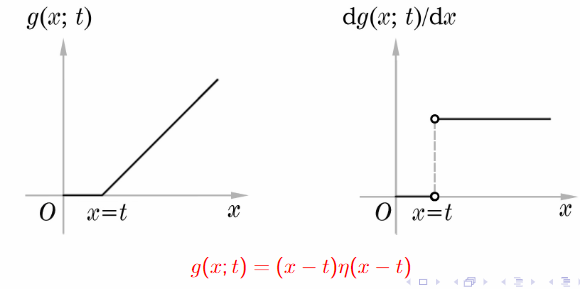解析：题目的物理意义，$\frac{\mathrm{d}^{2} g}{\mathrm{d} x^{2}}$代表加速度，在质点所加的力，这个力旨在x=t这样的时刻给了一个脉冲性的力，总量：积分后等于1.初始位置是0，加了力以后才开始运动。

有了EX19.4现在就可以求解下面的问题了
$\begin{array}{ll}{\frac{\mathrm{d}^{2} y}{\mathrm{d} x^{2}}=f(x)} & {x>0} \\ {y(0)=0} & {y^{\prime}(0)=0}\end{array}$
解答：因为$f(x)=\int_{0}^{\infty} f(t) \delta(x-t) \mathrm{d} t$，所以根据现行常微分方程解的叠加性，有（形式）解
$y(x)=\int_{0}^{\infty} g(x ; t) f(t) \mathrm{d} t=\int_{0}^{x}(x-t) f(t) \mathrm{d} t$
常微分方程的初值问题
EX19.5 常微分方程的初值问题
$\frac{\mathrm{d}^{2} g}{\mathrm{d} x^{2}}+k^{2} g(x ; t)=\delta(x-t) \quad x, t>0$
$\left.g\right|_{x=0}=0 \quad\left.\frac{\mathrm{d} g}{\mathrm{d} x}\right|_{x=0}=0$

能否直接写出非齐次常微分方程的通解？
当$x \neq t$时，方程的非齐次项为0
求出区间$(0,t)$内既满足齐次方程、又满足齐次初始条件的解…一定为零解
写出区间$(t,\infty)$内齐次微分方程的解
由$x=t$点处的连续性的要求定出Green函数
区间$(0,t)$内
$\frac{\mathrm{d}^{2} g}{\mathrm{d} x^{2}}+k^{2} g=0$
$\left.g\right|_{x=0}=0$
$\left.\frac{\mathrm{d} g}{\mathrm{d} x}\right|_{x=0}=0$
在$x=t$点
$g\left.(x ; t)\right|_{t-0} ^{t+0}=0$
$\left.\frac{d g}{d x}\right|_{t-0} ^{t+0}=1$
区间$(t,\infty)$
$\frac{d^{2} g}{d x^{2}}+k^{2} g=0$
解：$g(x ; t)=[C(t) \sin k x+D(t) \cos k x] \eta(x-t)$
$g(x ; t)$连续  $\left.\frac{\mathrm{d} g(x ; t)}{\mathrm{d} x}\right|_{t-0} ^{t+0}=1$
$C(t)=\frac{1}{k} \cos k t \qquad D(t)=-\frac{1}{k} \sin k t$
$g(x ; t)=\frac{1}{k} \sin k(x-t) \eta(x-t)$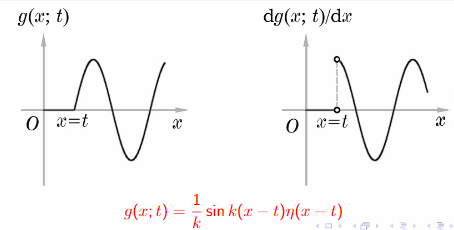思考：现在能否写出如下非齐次方程的通解
$\frac{\mathrm{d}^{2} g}{\mathrm{d} x^{2}}+k^{2} g(x ; t)=\delta(x-t) \quad x, t>0$

也能将上例的解用于求解非齐次方程初值问题
$\frac{\mathrm{d}^{2} y}{\mathrm{d} x^{2}}+k^{2} y(x)=f(x) \quad x>0$
$y(0)=0 \quad y^{\prime}(0)=0$
** $y(x)=\frac{1}{k} \int_{0}^{x} f(t) \sin k(x-t) \mathrm{d} t$
Remarks
对于一般的常微分方程初值问题的Green函数
$\frac{d}{d x}\left[p(x) \frac{d g(x ; t)}{d x}\right]+q(x) g(x ; t)=\delta(x-t) \quad x, t>0$
$g(0 ; t)=0 \quad\left.\frac{d g(x ; t)}{d x}\right|_{x=0}=0$
且相应的齐次微分方程无奇点

$g(x ; t)$在$x<t$时一定为0
$g(x ; t)$在$x=t$时一定连续
$\left.\frac{\mathrm{d} g(x ; t)}{\mathrm{d} x}\right|_{t-0} ^{t+0}=\frac{1}{p(t)}$

常微分方程的边值问题
EX19.6 求解常微分方程的边值问题
$\frac{d^{2} g}{d x^{2}}=\delta(x-t) \quad a<x, t<b$
$g(a ; t)=0 \quad g(b ; t)=0$
微分方程和EX19.4相同，故有相同的通解
$g(x ; t)=(x-t) \eta(x-t)+\alpha(t) x+\beta(t)$
$a \alpha(t)+\beta(t)=0 \quad b-t+b \alpha(t)+\beta(t)=0$
$\alpha(t)=-\frac{b-t}{b-a} \quad \beta(t)=\frac{a(b-t)}{b-a}$
$g(x ; t)=(x-t) \eta(x-t)-\frac{b-t}{b-a}(x-a)$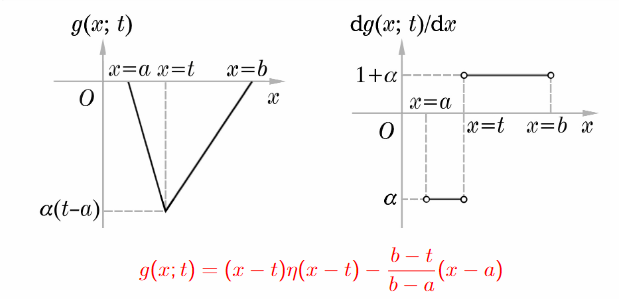问题：
本例中的Green函数$g(x ; t)$是否仍然满足
$g(x ; t)$在$x=t$点连续
$\frac{\mathrm{d} g(x ; t)}{\mathrm{d} x}$在$x=t$点不连续$\left.\frac{d g(x ; t)}{d x}\right|_{t-0} ^{t+0}=1$


展开全文• ## Delta函数的性质

千次阅读 2020-06-11 16:33:17
1. 定义性质：函数是通过其积分值来定义的 其中的积分为正向积分，对于任意在处连续的函数 2. 微分性质：采用分步积分可以建立恒等式 证明： 3. 偶函数性质： 4. 缩放性质： 证明：令，则有 5....
pdf文件

1. 定义性质：函数是通过其积分值来定义的

其中的积分为正向积分，对于任意在处连续的函数

2. 微分性质：采用分步积分可以建立恒等式

证明：

3. 偶函数性质：

4. 缩放性质：

证明：令，则有

5. 高级缩放性质：将函数作为函数的参量，和都是连续并连续可微的

6. 多参量零点性质：函数参量有多个零点，其缩放性质为

7. 多参数零点微分性质

参考文献：

 Tank, 2009, The Dirac Delta: Properties and Representations.

展开全文• 多元数量值函数积分 多元数量值函数的积分: 设(Ω)(\Omega)(Ω)表示一个有界的几何形体, 它是可度量的(即可求长或可求面积或可求体积), 函数fff是定义在(Ω)(\Omega)(Ω)上的有界数量值函数.将Ω\OmegaΩ任意地划分...
多元函数积分学多元数量值函数积分积分存在的条件和性质直角坐标系二重积分的计算法三重积分的计算含参变量的积分与反常重积分第一型线积分与面积分第二型线积分与面积分第二型线积分:第二型面积分Green公式平面曲线积分与路径无关的等价条件:
多元数量值函数积分

多元数量值函数的积分: 设$(\Omega)$表示一个有界的几何形体, 它是可度量的(即可求长或可求面积或可求体积), 函数$f$是定义在$(\Omega)$上的有界数量值函数.将$\Omega$任意地划分为n个小部分$(\Delta \Omega_k),k=1,2,3...,n$用$\Delta \Omega_k$表示$(\Delta \Omega_k)$的度量.任取点$M_k\in(\Delta \Omega_k)$,作乘积$f(M_k)\Delta \Omega_k,\ k=1,2,3,...,n$
作和式$\sum_{k=1}^{n}f(M_k)\Delta \Omega _k$
若不论$(\Omega)$如何划分, 点$M_k$在$(\Delta\Omega_k)$中如何选取, 当所有$(\Delta\Omega_k)$的直径的最大值$d\to0$时, 上述和式都趋于同一常数, 那么称函数$f$在$(\Omega)$上可积, 且称此常数为多元数量值函数$f$在$(\Omega)$上的积分, 记作$\int_{(\Omega)}f(M)d\Omega=\lim_{d\to 0}\sum_{k=1}^n f(M_k)\Delta \Omega_k$
其中$(\Omega)$称为积分域, $f$称为被积函数, $f(M)d\Omega$称为被积式或积分微元.
如果$\Omega$是$xOy$平面上的区域$(\sigma)$, 那么$f$就是定义在$(\sigma)$上的二元函数, $\Delta \Omega_k$就是子区域的面积$\Delta\sigma_k$, 从而积分式可以写成$\int _{(\Omega)}f(M)d\Omega=\lim_{d\to 0}\sum_{k=1}^n f(\mu_k,\eta_k)\Delta \sigma_k$称为$f$在区域$(\sigma)$上的二重积分, 其中$(\xi_k,\eta_k)$就是点$M_k$的直角坐标. 为了明确显示二重积分有两个独立的积分变量, 我们常用两个积分符号把二重积分表示为$\iint_{(\sigma)}f(x,y)d\sigma=\lim_{d\to 0}\sum_{k=1}^n f(\mu_k,\eta_k)\Delta \sigma_k$
其中$(\sigma)$是二重积分的积分域, $d\sigma$称为面积微元
如果$(\Omega)$为一条平面(或空间)的曲线弧段$(C)$, 那么$f$就是定义在弧段$(C)$上的二元(或三元)函数, $\Delta \Omega_k$就是子弧段的弧长$\Delta s_k$, 于是积分式可具体写成$\int_{(C)}f(x,y)ds=\lim_{k=1}^nf(\xi_k,\eta_k)\Delta s_k$
称为$f$在曲线段$(C)$上对弧长的曲线积分, 也称第一型线积分
如果$(\Omega)$为三维空间的区域(V), 那么f就是定义在(V)上的三元函数, $\Delta \Omega_k$就是子区域的体积$\Delta V_k$. 通常使用三个积分符号来表示$\iiint_{(V)}f(x,y,z)dV=\lim_{d\to 0}\sum_{k=1}^n f(\xi_k,\eta_k,\zeta_k)\Delta V_k$
称为$f$在区域$(V)$上的三重积分, 其中$(\xi_k,\eta_k,\zeta_k)$为点$M_k$的直角坐标, $(V)$使三重积分的积分域, $dV$称为体积微元.

积分存在的条件和性质

不论$(\Omega)$如何划分, 点$M_k$在$(\Delta\Omega_k)$中如何选取, 当所有$(\Delta\Omega_k)$的直径的最大值$d\to0$时, 上述和式都趋于同一常数, 那么称函数$f$在$(\Omega)$上可积. 可以证明, 若$(\Omega)$是有界笔记且可度量, $f\in C((\Omega))$, 则$f$在$(\Omega)$上一定可积.
线性性质
对积分域的可加性
积分不等式
中值定理: 设$f\in C((\Omega)),(\Omega)$为一有界连通闭集, 则在$(\Omega)$上至少存在一点P, 使$\int_{(\Omega)}f(M)d\Omega=f(P)\Omega$

直角坐标系二重积分的计算法

x型区域: 设$(\sigma)=\{(x,y)\ |\ a\leq x\leq b,y_1(x)\leq x\leq y_2(x)\}$,

三重积分的计算

球面坐标: 直角坐标到球面坐标的变换公式为$x=r\sin \varphi \cos\theta\\ y=r\sin\varphi\sin \theta\\z=r\cos \varphi\\ r\geq0,0\leq\varphi\leq\pi,0\leq\theta\leq2\pi$
体积微元是$\frac{\partial(x,y,z)}{\partial(r,\varphi,\theta)}=r^2\sin\varphi drd\varphi d\theta$
例题:

求曲面$(x^2+y^2+z^2)^2=a^3z(a>0)$所围立体体积.
求$I=\iiint_\Omega\sqrt{1-\frac{x^2}{a^2}-\frac{y^2}{b^2}-\frac{z^2}{c^2}}dV, \Omega: \frac{x^2}{a^2}+\frac{y^2}{b^2}+\frac{z^2}{c^2}\leq1$
分析: 可利用球面坐标, 设$x=ar\sin\varphi \cos\theta,y=br\sin\varphi\sin\theta, z=cr\cos\varphi$
求$x=\sqrt{y-z^2}, \frac{1}{2}\sqrt y=x, y=1$所围成的立体体积.
分析: 要点在于如何切割和计算范围. 本题可以在$x=1/2$处切割, 恰好分为两个可计算的区域. 另外, 求变量范围时,  注意某坐标为应为定值时, 直接带入计算范围即可.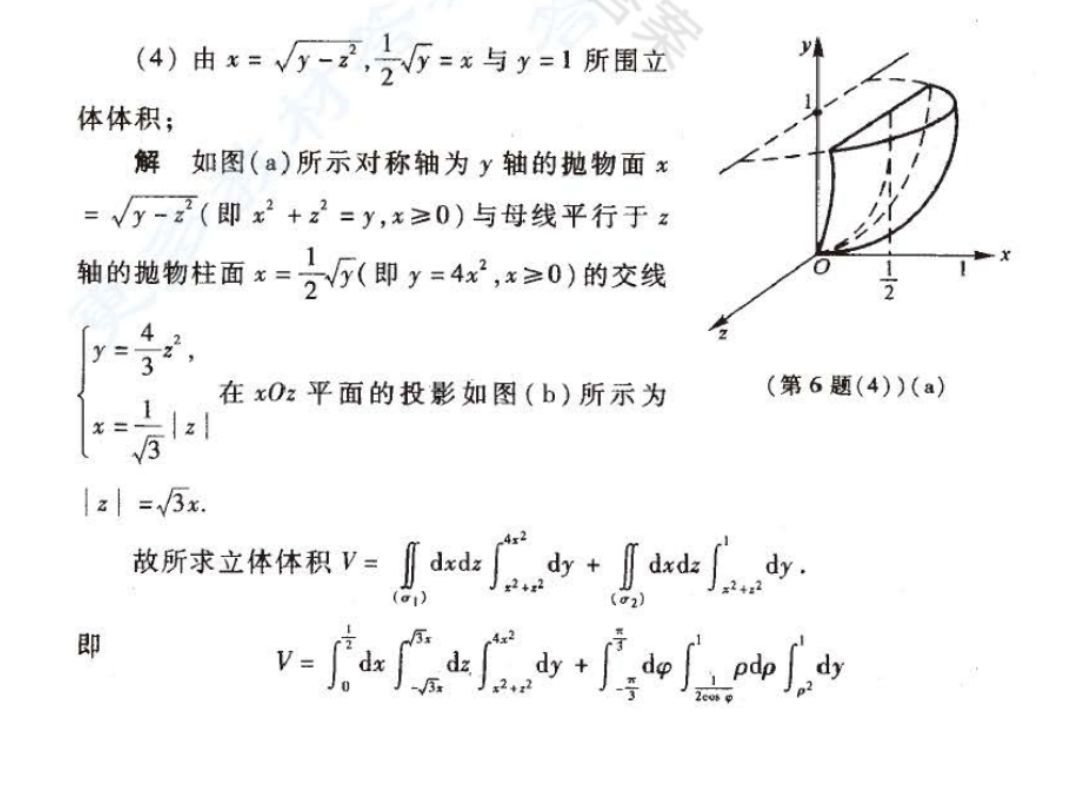由$\frac{x^2}{a^2}+\frac{y^2}{b^2}+\frac{z^2}{c^2}=-1, \frac{x^2}{a^2}+\frac{y^2}{b^2}=1$所围成的立体体积.
分析: 利用柱面坐标, 只计算上半部分即可
计算$\iiint_{(V)}|\sqrt{x^2+y^2+z^2}-1|dV, (V)$由$z=\sqrt{x^2+y^2},z=1$围成.
分析: 讨论范围, 分为球内和球外, 分别计算范围即可
计算$\iiint_{(V)}(x+y+z)^2dV, (V)$为椭球体$\frac{x^2}{a^2}+\frac{y^2}{b^2}+\frac{z^2}{c^2}\leq1$.
分析: 首先应去除积分为0的项, 在计算剩余项的积分, 用到椭圆面积公式.

含参变量的积分与反常重积分

含参变量积分定义: 记$D=[a,b]\times[c,d]$. 如果$f\in C(D),$那么对任一固定$y\in[c,d]$,积分$F(y)=\int_a^b f(x,y)dx$存在, 且将随y的变化而变化, 我们称上积分为含参变量y的积分, 它是参变量y的函数.
连续性: 若$f\in C(D)$, 则$F(y)=\int_a^b f(x,y)dx$在区间$[c,d]$连续.
可导性: 若$f\in C(D),f_y\in C(D)$, 则$F(y)=\int_a^b f(x,y)dx$在$[c,d]$上有连续的导数, 且求导和积分可交换顺序. 即$F'(y)=\frac{d}{dy}\int_a^b f(x,y)dx=\int_a^b \frac{\partial f(x,y)}{\partial y}dx$
积分顺序交换性: 若$f\in C(D),$ 则$F(y)=\int_a^bf(x,y)dx在[c,d]可积\\[2ex] G(x)=\int_c^df(x,y)dy在[a,b]可积.$
且$\int_c^b(\int_a^bf(x,y)dx)dy=\int_a^b(\int_c^df(x,y)dy)dx$
设$f(x,y)\in C(D). x_i(y)\in C[c,d],i=1,2$,且其值域均为$[a,b]$.则$F(y)=\int_{x_1(y)}^{x_2(y)}f(x,y)dx$必在$[c,d]$上连续
若$f(x,y),f_y(x,y)$均在$D$上连续, $x_1(y),x_2(y)$的值域均为$[a,b]$, 且他们都在$[c,d]$上可导, 则$F(y)=\int_{x_1(y)}^{x_2(y)}f(x,y)dx$也在$[c,d]$上可导. 且有$F'(y)=\int_{x_1(y)}^{x_2(y)}f_y(x,y)dx+f[x_2(y),y]x'_2(y)-f[x_1(y),y]x'_1(y).$
例题:

计算积分$\int_0^1\frac{x^b-x^a}{\ln x}dx,(a,b)>0$
分析: 直接求积分不好求, 考虑化成二重积分. $x^y$对y的导数为$x^y/\ln x$, 原式可化为$\int_0^1dx\int_a^bx^ydy$,再交换积分顺序计算即可.
计算积分$I=\int_0^1\frac{\ln{(1+x)}}{1+x^2}dx$.

第一型线积分与面积分

第一型线积分: 曲线积分的值与积分路径( C )的方向无关, 我们把这种曲线积分称为对弧长的线积分, 也称第一型线积分.
计算公式: 设有一有界简单光滑曲线©, 其参数方程为$x=x(t),y=y(t),z=z(t).(\alpha\leq t\leq \beta)$
若函数$f(x,y,z)$在C上连续, 则$\int_{ (C) }f(x,y,z)ds=\int_\alpha^\beta f[x(t),y(t),z(t)]\sqrt{\dot x^2(t)+\dot y^2(t)+\dot z^2(t)}dt$

第二型线积分与面积分
第二型线积分:

定义: 设L为xoy平面内从A到B的一条又向光滑弧, 在L上定义了一个向量函数$\vec F(x,y)=(P(x,y),Q(x,y))$,若对L的任意分割和在局部弧段上取任意点, 极限$\lim_{\lambda\to 0}\sum_{k=1}^n [P(\xi_k,\eta_k)\Delta x+Q(\xi_k,\eta_k)\Delta y]=\int_L P(x,y)dx +Q(x,y)dy$都存在, 则称此极限为函数$\vec F(x,y)$在又向曲线弧L上对坐标的曲线积分, 或称第二类曲线积分. 其中$P(x,y),Q(x,y)$称为被积函数, L称为积分弧段或积分曲线.

第二型面积分

定义: 设在向量场$\pmb A(M)$中有一可求面积的有向曲面, 指定它的一侧. 把曲面任意划分成n小片$(\Delta S_1),(\Delta S_2)...(\Delta S_n)$,任取一点$\pmb A(M_k)\cdot \pmb e_n(M_k)\Delta S_k \ (k=1,2,...n)$
其中$\pmb e_n$为曲面在点$M_k$处指向给定测的单位法向量.$\Delta S_k$表示微元面积.
作和式$\sum_{k=1}^n\pmb A(M_k)\cdot\pmb e_n (M_k)\Delta S_k$
如果不论曲面$S$如何划分, 点$M_k$怎样选取, 当个小曲面直径最大值趋于零时上述和式都趋于同一常数, 则称此极限值为向量场$\pmb A(M)$沿有向曲面(S)的第二型曲面积分, 简称第二型面积分, 记作$\iint_{(S)}\pmb A(M)\cdot \pmb{dS}=\lim_{d\to 0}\sum_{k=1}^n\pmb A(M_k)\cdot\pmb e_n (M_k)\Delta S_k$
其中$\pmb{dS}=\pmb e_ndS$称为曲面面积微元向量.

Green公式

单连通域: 若区域$\sigma$内任意一条闭曲线的内部全部属于$\sigma$, 或者说$\sigma$内任意一闭曲线均可在$\sigma$内连续变形缩小成$\sigma$内的一点, 则称$\sigma$是一单连通域, 否则称为复连通域.
格林公式: 设平面内有界比区域$\sigma$由一条分段光滑的简单闭曲线所围成, $\sigma$的边界即为$C$, 函数$P,Q\in C^{(1)}((\sigma))$,则下述公式成立
$\iint_{(\sigma)}(\frac{\partial Q}{\partial x}-\frac{\partial P}{\partial y})d\sigma=\oint_{(+C)}P(x,y)dx+Q(x,y)dy$
例题

计算$\int_L(x^2+3y)dx+(y^2-x)dy$, 其中L是上半圆周$y=\sqrt{4x-x^2}$从$(0,0)$到$(4,0)$.
分析: 为使用格林公式, 可将半圆补全在减去.
计算$\int_L\frac{xdy-ydx}{x^2+y^2}$, 其中L是无重点且不过原点的分段光滑正向闭曲线.
分析: 需讨论图形是否包含远点的情况. 当不包含原点时, 直接为0, 包含原点时, 应围绕远点作圆域, 令该圆域半径趋于0, 计算此时的第二型线积分, 直接用L正向积分减去半径趋于0的圆域积分得到闭区域的积计算得零, 再计算圆域积分.

平面曲线积分与路径无关的等价条件:

定理: 设D为单连通区域, 函数P(x,y),Q(x,y)再D内具有一阶连续偏导数, 则以下四个条件等价:

沿D中任意光滑闭曲线, 有$\oint_L Pdx+Qdy=0$
对D中任一分段光滑曲线$L_1$曲线积分$\int_LPdx+Qdy$与路径无关, 只与起止点有关.
$Pdx+Qdy$在D内有某一函数$u(x,y)$的全微分, 即$du(x,y)=Pdx+Qdy$
在D内每一点都有$\frac{\partial P}{\partial y}=\frac{\partial Q}{\partial x}$


展开全文• 二重积分  二重积分（f(x,y)f(x,y)f(x,y) 在 σ\sigmaσ 上的黎曼积分） ∬σf(x,y)dσ=lim⁡λ→0∑i=1nf(ξi,ηi)Δσi\...{i=1}^{n}{f(\xi_i,\eta_i)\Delta \sigma_i}}∬σ​f(x,y)dσ=λ→0lim​i=1∑n​f
二重积分
二重积分（$f(x,y)$ 在 $\sigma$ 上的黎曼积分） $\displaystyle\iint_{\sigma}{f(x,y)\mathrm{d}\sigma}=\displaystyle\lim_{\lambda \to 0}{\displaystyle\sum_{i=1}^{n}{f(\xi_i,\eta_i)\Delta \sigma_i}}$
绝对值不等式 $\left|\displaystyle\iint_{\sigma}{f(x,y)\mathrm{d}\sigma}\right| \leq \displaystyle\iint_{\sigma}{|f(x,y)|\mathrm{d}\sigma}$
二重积分中值定理 若 $f(x,y)$ 在有界闭区域 $\sigma$ 上连续，则至少存在一点 $P(x^*,y^*) \in \sigma$，使得 $\displaystyle\iint_{\sigma}{f(x,y)\mathrm{d}\sigma}=f(x^*,y^*)\sigma$，其中 $f(x^*,y^*)=\dfrac1\sigma\displaystyle\iint_{\sigma}{f(x,y)\mathrm{d}\sigma}$ 称为 $f(x,y)$ 在 $\sigma$ 上的平均值。
推论 若 $m \leq f(x,y) \leq M$，则 $m\sigma \leq \displaystyle\iint_{\sigma}{f(x,y)\mathrm{d}\sigma} \leq M\sigma$。
二重积分转化为累次积分 \begin{aligned}\displaystyle\iint_{\sigma}{f(x,y)\mathrm{d}\sigma}&=\displaystyle\iint_{\sigma}{f(x,y)\mathrm{d}x\mathrm{d}y} \\ &=\displaystyle\int_{a}^{b}{\mathrm{d}x}\displaystyle\int_{\varphi_1(x)}^{\varphi_2(x)}{f(x,y)\mathrm{d}y}=\displaystyle\int_{c}^{d}{\mathrm{d}y}\displaystyle\int_{\psi_1(y)}^{\psi_2(y)}{f(x,y)\mathrm{d}x}\end{aligned} 二重积分的极坐标变换 $\begin{cases}x=r\cos\theta \\ y=r\sin\theta\end{cases}$ \begin{aligned}\displaystyle\iint_{\sigma}{f(x,y)\mathrm{d}\sigma}&=\displaystyle\iint_{\sigma}{f(r\cos\theta,r\sin\theta)r\mathrm{d}r\mathrm{d}\theta} \\ &=\displaystyle\int_{\alpha}^{\beta}{\mathrm{d}\theta}\displaystyle\int_{r_1(\theta)}^{r_2(\theta)}{f(r\cos\theta,r\sin\theta)r\mathrm{d}r}=\displaystyle\int_{r_1}^{r_2}{\mathrm{d}r}\displaystyle\int_{\theta_1(r)}^{\theta_2(r)}{f(r\cos\theta,r\sin\theta)r\mathrm{d}\theta}\end{aligned} $\Longleftarrow \mathrm{d}\sigma=\dfrac12(r+\mathrm{d}r)^2\sin\mathrm{d}\theta-\dfrac12r^2\sin\mathrm{d}\theta=r\mathrm{d}r\sin\mathrm{d}\theta+\dfrac12(\mathrm{d}r)^2\sin\mathrm{d}\theta \sim r\mathrm{d}r\sin\mathrm{d}\theta \sim r\mathrm{d}r\mathrm{d}\theta$
二重积分的一般坐标变换（雅可比行列式） $\displaystyle\iint_{\sigma}{f(x,y)\mathrm{d}x\mathrm{d}y}=\displaystyle\iint_{\sigma}{g(u,v)|J|\mathrm{d}u\mathrm{d}v}$ $J=\dfrac{\partial(x,y)}{\partial(u,v)}=\begin{vmatrix}\dfrac{\partial x}{\partial u} & \dfrac{\partial x}{\partial v} \\ \dfrac{\partial y}{\partial u} & \dfrac{\partial y}{\partial v}\end{vmatrix}=\dfrac{1}{\dfrac{\partial(u,v)}{\partial(x,y)}}=\begin{vmatrix}\dfrac{\partial u}{\partial x} & \dfrac{\partial u}{\partial y} \\ \dfrac{\partial v}{\partial x} & \dfrac{\partial v}{\partial y}\end{vmatrix}^{-1}$
三重积分
三重积分（密度函数 $f(x,y,z)$ 在空间立体 $V$ 上的质量） $M=\displaystyle\iiint_{V}{f(x,y,z)\mathrm{d}V}=\displaystyle\lim_{\lambda \to 0}{\displaystyle\sum_{i=1}^{n}{f(\xi_i,\eta_i,\zeta_i)\Delta V_i}}$
三重积分转化为累次积分（投影法） \begin{aligned}\displaystyle\iiint_{V}{f(x,y,z)\mathrm{d}V}&=\displaystyle\iiint_{V}{f(x,y,z)\mathrm{d}x\mathrm{d}y\mathrm{d}z} \\ &=\displaystyle\int_{a}^{b}{\mathrm{d}x}\displaystyle\int_{\varphi_1(x)}^{\varphi_2(x)}{\mathrm{d}y}\displaystyle\int_{z_1(x,y)}^{z_2(x,y)}{f(x,y,z)\mathrm{d}z}=\displaystyle\int_{c}^{d}{\mathrm{d}y}\displaystyle\int_{\psi_1(y)}^{\psi_2(y)}{\mathrm{d}x}\displaystyle\int_{z_1(x,y)}^{z_2(x,y)}{f(x,y,z)\mathrm{d}z} \\ &=\displaystyle\iint_{\sigma_{zx}}{\mathrm{d}\sigma}\displaystyle\int_{y_1(x,z)}^{y_2(x,z)}{f(x,y,z)\mathrm{d}y} \\ &=\displaystyle\iint_{\sigma_{yz}}{\mathrm{d}\sigma}\displaystyle\int_{x_1(y,z)}^{x_2(y,z)}{f(x,y,z)\mathrm{d}x}\end{aligned} 三重积分转化为累次积分（截割法） \begin{aligned}\displaystyle\iiint_{V}{f(x,y,z)\mathrm{d}V}&=\displaystyle\iiint_{V}{f(x,y,z)\mathrm{d}x\mathrm{d}y\mathrm{d}z} \\ &=\displaystyle\int_{e}^{f}{\mathrm{d}z}\displaystyle\iint_{D_z}{f(x,y,z)\mathrm{d}\sigma}=\displaystyle\int_{e}^{f}{\mathrm{d}z}\displaystyle\iint_{D_z}{f(x,y,z)\mathrm{d}x\mathrm{d}y} \\ &=\displaystyle\int_{c}^{d}{\mathrm{d}y}\displaystyle\iint_{D_y}{f(x,y,z)\mathrm{d}\sigma} \\ &=\displaystyle\int_{a}^{b}{\mathrm{d}x}\displaystyle\iint_{D_x}{f(x,y,z)\mathrm{d}\sigma}\end{aligned} 三重积分的柱坐标变换 $\begin{cases}x=r\cos\theta \\ y=r\sin\theta \\ z=z\end{cases}$ $\displaystyle\iiint_{V}{f(x,y,z)\mathrm{d}V}=\displaystyle\iiint_{V}{f(r\cos\theta,r\sin\theta,z)r\mathrm{d}r\mathrm{d}\theta\mathrm{d}z}=\displaystyle\iint_{\sigma}{r\mathrm{d}r\mathrm{d}\theta}\displaystyle\int_{z_1(r,\theta)}^{z_2(r,\theta)}{f(r\cos\theta,r\sin\theta,z)\mathrm{d}z}$ 三重积分的球坐标变换 $\begin{cases}x=\rho\sin\varphi\cos\theta \\ y=\rho\sin\varphi\sin\theta \\ z=\rho\cos\varphi\end{cases}$ $\displaystyle\iiint_{V}{f(x,y,z)\mathrm{d}V}=\displaystyle\iiint_{V}{f(\rho\sin\varphi\cos\theta,\rho\sin\varphi\sin\theta,\rho\cos\varphi)\rho^2\sin\varphi\mathrm{d}\rho\mathrm{d}\varphi\mathrm{d}\theta}$ $\Longleftarrow \mathrm{d}V=\rho\tan\mathrm{d}\varphi \cdot \rho\sin\varphi\tan\mathrm{d}\theta \cdot \mathrm{d}\rho=\rho^2\sin\varphi\mathrm{d}\rho\tan\mathrm{d}\varphi\tan\mathrm{d}\theta \sim \rho^2\sin\varphi\mathrm{d}\rho\mathrm{d}\varphi\mathrm{d}\theta$
三重积分的一般坐标变换（雅可比行列式） $\displaystyle\iiint_{V}{f(x,y,z)\mathrm{d}x\mathrm{d}y\mathrm{d}z}=\displaystyle\iiint_{V}{g(u,v,w)|J|\mathrm{d}u\mathrm{d}v\mathrm{d}w}$ $J=\dfrac{\partial(x,y,z)}{\partial(u,v,w)}=\begin{vmatrix}\dfrac{\partial x}{\partial u} & \dfrac{\partial x}{\partial v} & \dfrac{\partial x}{\partial w} \\ \dfrac{\partial y}{\partial u} & \dfrac{\partial y}{\partial v} & \dfrac{\partial y}{\partial w} \\ \dfrac{\partial z}{\partial u} & \dfrac{\partial z}{\partial v} & \dfrac{\partial z}{\partial w}\end{vmatrix}=\dfrac{1}{\dfrac{\partial(u,v,w)}{\partial(x,y,z)}}=\begin{vmatrix}\dfrac{\partial u}{\partial x} & \dfrac{\partial u}{\partial y} & \dfrac{\partial u}{\partial z} \\ \dfrac{\partial v}{\partial x} & \dfrac{\partial v}{\partial y} & \dfrac{\partial v}{\partial z} \\ \dfrac{\partial w}{\partial x} & \dfrac{\partial w}{\partial y} & \dfrac{\partial w}{\partial z}\end{vmatrix}^{-1}$
第一类线面积分
第一类曲线积分（弧长积分） $\displaystyle\int_{\Gamma}{f(P)\mathrm{d}s}=\displaystyle\lim_{\lambda \to 0}{\displaystyle\sum_{i=1}^{n}{f(P_i)\Delta s_i}}=\displaystyle\int_{\alpha}^{\beta}{f(x(t),y(t),z(t))\sqrt{x'^2(t)+y'^2(t)+z'^2(t)}\mathrm{d}t}$ \begin{aligned}\Longleftarrow \mathrm{d}s&=\sqrt{[x(t+\mathrm{d}t)-x(t)]^2+[y(t+\mathrm{d}t)-y(t)]^2+[z(t+\mathrm{d}t)-z(t)]^2} \\ &=\sqrt{x'^2(\xi_1)+y'^2(\xi_2)+z'^2(\xi_3)}\mathrm{d}t \ (t<\xi_1,\xi_2,\xi_3
平面曲线弧长积分 $\displaystyle\int_{\Gamma}{f(x,y)\mathrm{d}s}=\displaystyle\int_{\alpha}^{\beta}{f(x(t),y(t))\sqrt{x'^2(t)+y'^2(t)}\mathrm{d}t}$
（1） $\Gamma:y=\varphi(x),x \in [a,b] \Longrightarrow \displaystyle\int_{\Gamma}{f(x,y)\mathrm{d}s}=\displaystyle\int_{a}^{b}{f(x,\varphi(x))\sqrt{1+\varphi'^2(x)}\mathrm{d}x}$；
（2） $\Gamma:x=\psi(y),y \in [c,d] \Longrightarrow \displaystyle\int_{\Gamma}{f(x,y)\mathrm{d}s}=\displaystyle\int_{c}^{d}{f(\psi(y),y)\sqrt{1+\psi'^2(y)}\mathrm{d}y}$；
（3） $\Gamma:r=r(\theta),\theta \in [\alpha,\beta] \Longrightarrow \displaystyle\int_{\Gamma}{f(x,y)\mathrm{d}s}=\displaystyle\int_{\alpha}^{\beta}{f(r\cos\theta,r\sin\theta)\sqrt{r^2(\theta)+r'^2(\theta)}\mathrm{d}\theta}$。
第一类曲面积分 $\displaystyle\iint_{S}{f(P)\mathrm{d}S}=\displaystyle\lim_{\lambda \to 0}{\displaystyle\sum_{i=1}^{n}{f(P_i)\Delta S_i}}$
（1） $S:z=z(x,y),(x,y) \in \sigma_{xy} \Longrightarrow \displaystyle\iint_{S}{f(x,y,z)\mathrm{d}S}=\displaystyle\iint_{\sigma_{xy}}{f(x,y,z(x,y))\sqrt{1+z_x'^2+z_y'^2}}\mathrm{d}\sigma$；
取面微元 $\mathrm{d}S$ 上一点 $P(x,y,z(x,y))$，则该点处法线的方向矢量 $\boldsymbol{n}=\pm \{z_x',z_y',-1\}$，记 $\gamma$ 为 $\boldsymbol{n}$ 与 $z$ 轴正方向的夹角，有 $\cos\gamma=\pm \dfrac{1}{\sqrt{1+z_x'^2+z_y'^2}}$，故 $\mathrm{d}S$ 在 $Oxy$ 平面上的投影面积 $\mathrm{d}\sigma=|\cos\gamma| \cdot \mathrm{d}S$，可得 $\mathrm{d}S=\dfrac{\mathrm{d}\sigma}{|\cos\gamma|}=\sqrt{1+z_x'^2+z_y'^2}\mathrm{d}\sigma$。
（2） $S:y=y(x,z),(x,z) \in \sigma_{zx} \Longrightarrow \displaystyle\iint_{S}{f(x,y,z)\mathrm{d}S}=\displaystyle\iint_{\sigma_{zx}}{f(x,y(x,z),z)\sqrt{1+y_x'^2+y_z'^2}}\mathrm{d}\sigma$；
（3） $S:x=x(y,z),$